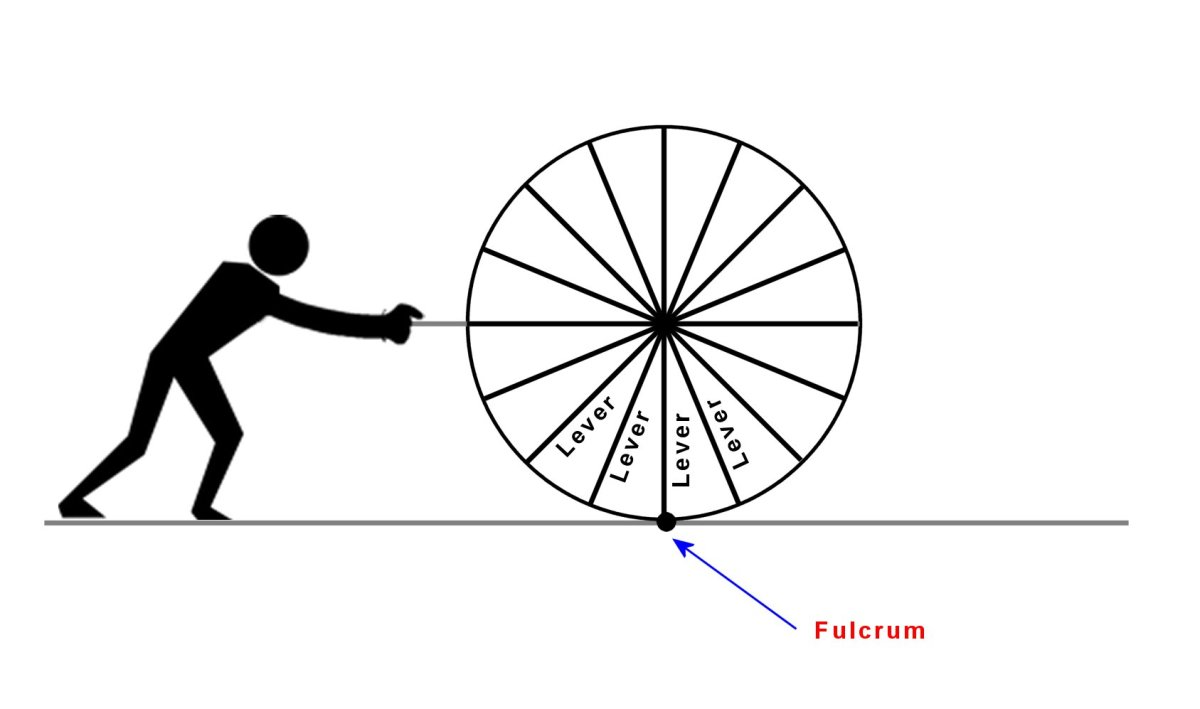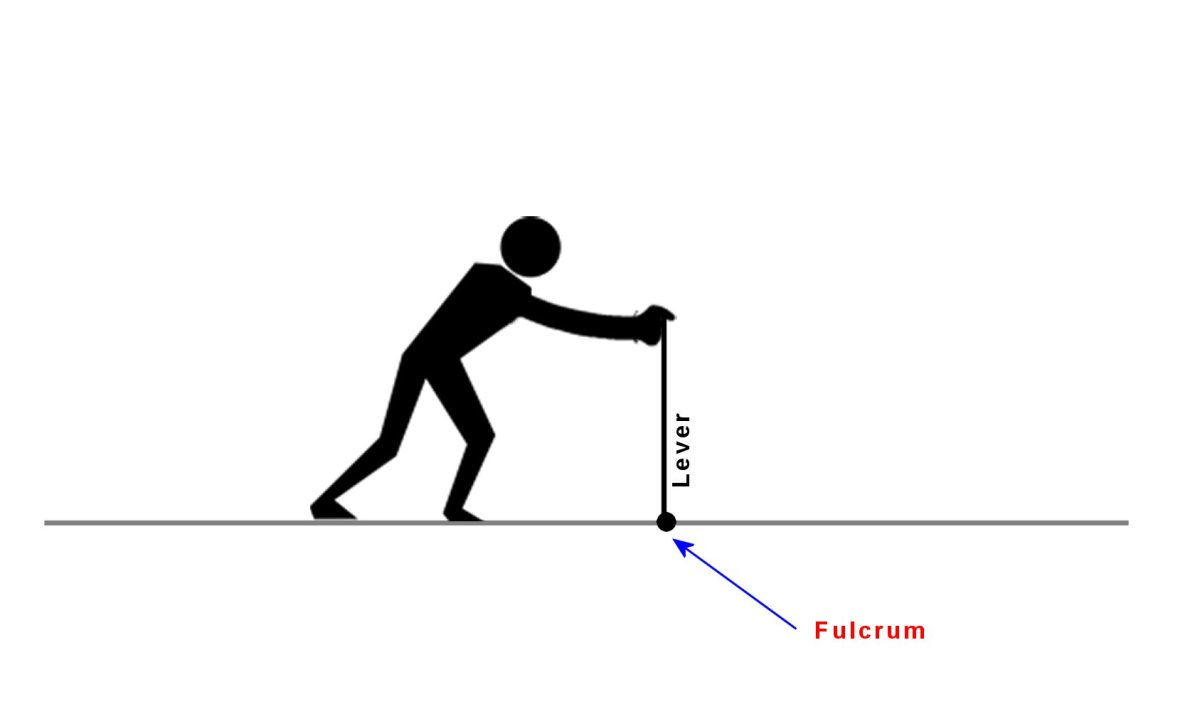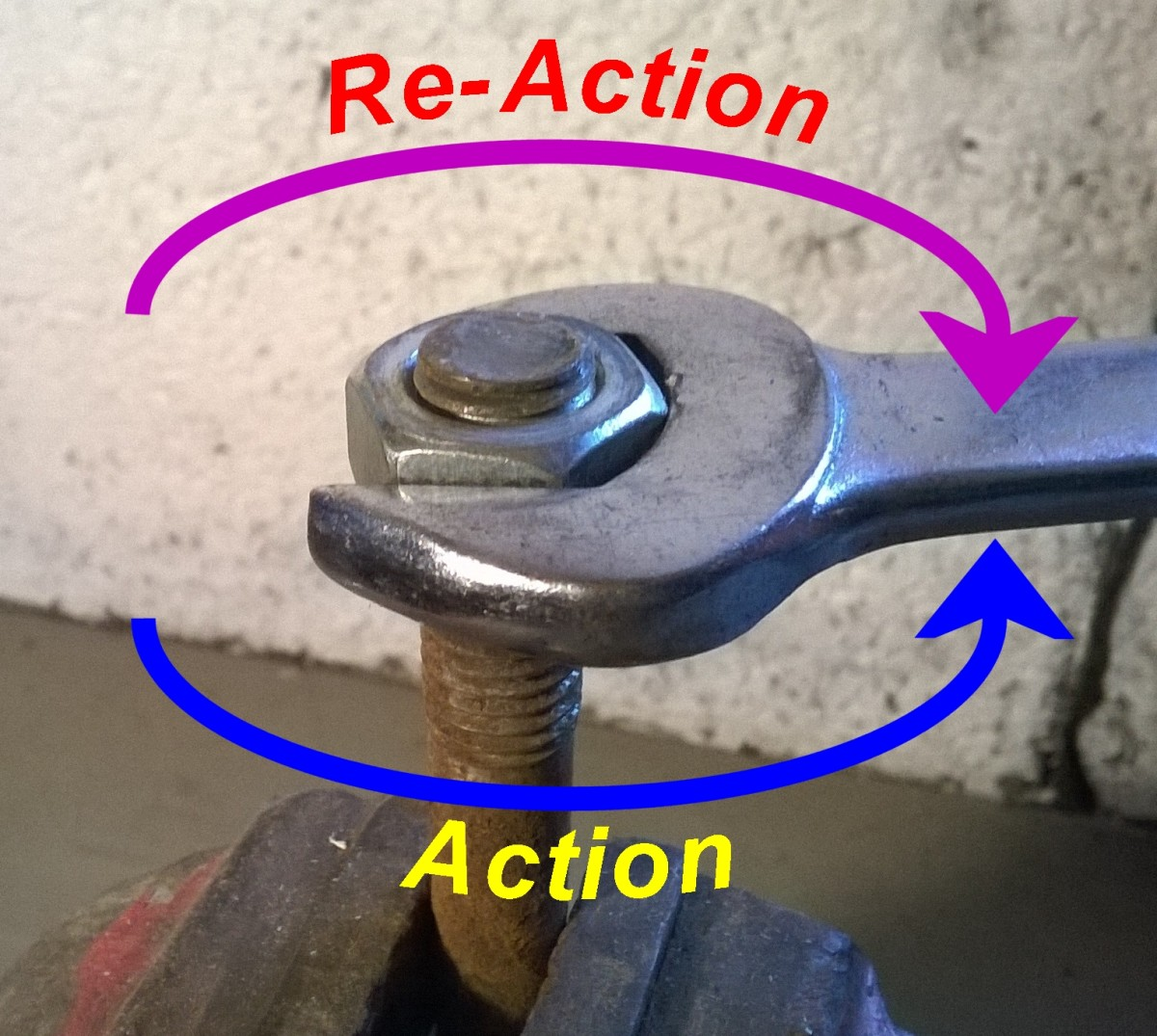# Simple Machines — How Do Wheels and Axles Work?

## The Wheel and Axle — One of the Six Classical Simple Machines

Wheels are everywhere in our modern technological society, but they have also been used since ancient times. The place you are most likely to see a wheel is on a vehicle or trailer, but wheels are used for a variety of other applications. They are widely used in machines in the form of gears, pulleys, bearings, rollers and hinges. The wheel relies on the lever to reduce friction.
The wheel and axle are one of the six classical simple machines defined by Renaissance scientists, which also includes the lever, the pulley, the wedge, the inclined plane and the screw.

Before you read this explanation which becomes a bit technical, it would be helpful to read another related article which explains the basics of mechanics.

Force, Mass, Acceleration and How to Understand Newton's Laws of Motion

## The History of the Wheel

Wheels were unlikely to have been invented by just one person, and probably developed in many civilizations independently over the millenia. We can only imagine how it happened. Maybe some bright spark noticed how easy it was to slide something over ground with rounded stone pebbles on it, or observed how easily tree trunks could be rolled, once cut down. The first "wheels" were probably rollers made from tree trunks and positioned under heavy loads. The problem with rollers is that they are long and heavy and have to be continually re-positioned under the load, so the axle had to be invented to hold a thinner disk, effectively a wheel, in place. Early wheels were likely made from stone or flat boards joined together into the form of a disk.

## Moment of a Force

To understand how wheels and levers work, we need to understand the concept of moment of a force. The moment of a force about a point is the magnitude of the force multiplied by the perpendicular distance from the point to the line of the force.

## Why Do Wheels Make it Easier to Push Things?

It all boils down to reducing friction. So imagine if you have a heavy weight resting on the ground. Newton's 3rd Law states that "For every action, there is an equal and opposite reaction". So when you try to push the load, the force transmits through the load to the surface it rests on. This is the action. The corresponding reaction is the force of friction acting backwards and is dependent on both the nature of the surfaces in contact and the weight of the load. This is known as static friction or stiction and applies to dry surfaces in contact. Initially the reaction matches the action in magnitude and the load doesn't move, but eventually if you push hard enough, the friction force reaches a limit and doesn't increase further. If you push harder, you exceed the limiting friction force and the load starts to slide. The force of friction however continues to oppose motion (it reduces a bit once motion starts), and if the load is very heavy and/or the surfaces in contact have a high coefficient of friction, it can be difficult to slide it.
Wheels eliminate this friction force by using leverage and an axle. They still need friction so that they can "push back" on the ground on which they roll, otherwise slippage occurs. This force however doesn't oppose motion or make it more difficult for the wheel to roll.

## A Wheel Behaves Like an Infinite Number of Levers

A solid wheel can be thought of as a series of levers with a different lever touching the ground as the wheel rolls (These aren't individual spokes, it's just a way of thinking of how the wheel works). Each lever pivots at the ground surface in the diagram below and the wheel is moved forward as it is pushed. Since the wheel doesn't slide, the friction force that previously existed when an object was being slid now disappears and so there's no opposing force to motion at the ground.

## A Wheel Acts Like a Series of LeversA solid wheel can be thought of as an infinite number of levers, each pivoting at the ground surface as the wheel rolls.© Eugene BrennanAt any moment, it's as if a single lever is being pushed at the top.© Eugene Brennan

## How Do Wheels Work?

### Analysis of Wheel Due to a Force at the Axle

This analysis applies to the example above where the wheel is subject to a force or effort F at the axle.

### Fig. 1

A force acts on the axle whose radius is d.

### Fig. 2

Two new equal but opposite forces are introduced where the wheel meets the surface. This technique of adding fictitious forces which cancel each other out is useful for solving problems.

### Fig. 3

When two forces act in opposite directions, the result is known as a couple and its magnitude is called the torque. In the diagram, the added forces result in a couple plus a force F where the wheel meets the surface. The magnitude of this couple is the force multiplied by the radius of the wheel.

So Torque Tw = Fd.

### Fig. 4

A lot is going on here! The blue arrows indicate the active forces, the purple the reactions. To make the diagram clearer, the couple consisting of the two forces F in the diagram above has been replaced by the torque Tw . This acts clockwise. Again Newton's third law comes into play and there is a limiting reactive torque Tr at the axle. This is due to friction caused by weight on the axle. Rust can increase the limiting value, lubrication reduces it. More about this later.

In reality things are more complicated and there is additional reaction due to the moment of the inertia of the wheels, but let's not complicate things and assume the wheels are weightless!

• The weight acting down on the wheel due to the weight of the cart is W.
• The reaction at the ground surface is Rn = W.
• The F shown in the diagram above acts forward at the ground. This is balanced by a force Ff acting backwards. If the wheel doesn't slip, this allows the levers we talked about earlier to pivot at the point of contact and turn.
• The force Ff has a limiting value of Ff = μRn. If F exceeds Ff, the wheel will start to slide.

## Active and Reactive Torques

An example of active and reactive torques is when you try to undo a nut which is rusted onto a bolt. You apply a torque with a wrench, but the rust binds the nut and acts against you. If you apply enough torque, you overcome the reactive torque which has a limiting value. If the nut is thoroughly seized up and you apply too much force, the bolt will wring.Undoing a nut. The limiting value of friction must be overcome to release the nut© Eugene Brennan

### Fig. 5

The two forces which produce the torque Tw are shown again. Now you can see this resembles a lever system as explained above. F acts over distance d, and the reaction at the axle is Fr.
The force F is magnified at the axle and is shown by the green arrow. Its magnitude is:

Fe = F(d/a)

Since the ratio of the wheel diameter to the axle diameter is large , ie d/a, the minimum force F required for movement is proportionately reduced. The wheel effectively works as a lever, magnifying the force at the axle, and overcoming the limiting value of the friction force Fr. Notice also for a given axle diameter a, if the wheel diameter is made bigger, Fe becomes larger. So it's easier to push something with big wheels than small wheels because there is a greater force at the axle to overcome friction.

## Which is Better, Big Wheels or Small Wheels?

Since

Torque = Force at Axle x Radius of Wheel

for a given force at the axle, the torque acting at the axle is bigger for bigger wheels. So friction at the axle is greatly overcome,and therefore it's easier to push something with bigger wheels. Also if the surface that the wheel rolls on isn't very flat, larger diameter wheels tend to bridge imperfections, which also reduces the effort required.

When a wheel is driven by an axle, since

Torque = Force at Axle x Radius of Wheel

therefore

Force at Axle = Torque / Radius of Wheel

So for a constant driving torque, smaller diameter wheels produce a greater tractive effort at the axle than larger wheels. This is the force which pushes a vehicle.

This content is accurate and true to the best of the author’s knowledge and is not meant to substitute for formal and individualized advice from a qualified professional.